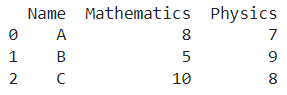# Creating An Empty Pandas DataFrame, Then Filling It

A DataFrame is the primary data structure of the Pandas library in Python and is commonly used for storing and working with tabular data.

A common operation that could be performed on such data is to create an empty dataframe and then fill it in order to work with the information in a better manner.

To start working with Pandas, we first need to import it:

``import pandas as pd``

## Running Example

Let us understand this operation with the help of an example. Consider the following DataFrame containing 3 students with names A, B, and C and their corresponding marks (out of 10) for two subjects, Mathematics and Physics.Code snippet for generating the above DataFrame :

``````import pandas as pd

# Dictionary for our data
data = {'Name' : ['A', 'B', 'C'], 'Mathematics' : [8, 5, 10], 'Physics' : [7, 9, 8]}

# DataFrame for the dictionary
df = pd.DataFrame(data)

# Printing the DataFrame
print(df)``````

Here, data is a dictionary we created to initialize the DataFrame. For this, we use the DataFrame() function of the Pandas library which takes the dictionary as an argument and returns the required DataFrame.

Now, let’s say we need to create this in a different manner without passing a dictionary as a parameter. We shall create an empty dataframe and then fill it. The resulting output would look like this :Let us look at different ways of performing this operation on a given DataFrame :

## 1. Using the loc property with a list

In this method, we use the loc property to fill data in a dataframe after creating an empty one.

We do this with the help of a for-loop. We use the iterator variable i to access the new row using square brackets.

We access the elements in the dictionary using square brackets and the keys for corresponding column labels. We then index the list using the iterator variable.

Let us take a look at the corresponding code snippet and generated output for this method:

``````# Importing pandas
import pandas as pd

# Dictionary for our data
data = {'Name' : ['A', 'B', 'C'], 'Mathematics' : [8, 5, 10], 'Physics' : [7, 9, 8]}

# Create an empty dataframe
df = pd.DataFrame(columns = ['Name', 'Mathematics', 'Physics'])

# Performing the operation
for i in range(3):
Â Â df.loc[i] = [data['Name'][i], data['Mathematics'][i], data['Physics'][i]]

# Printing the dataframe
print(df)``````

Output :## 2. Using the loc property with dictionaries

In this method, we use the loc property to fill data in a dataframe after creating an empty one. We do this with the help of a for-loop.

We use the iterator variable i to access the new row using square brackets. We assign to this new row a dictionary containing the elements for corresponding columns with keys as the appropriate column labels. We access the elements in the dictionary using square brackets and the keys for corresponding column labels.

We then index the list using the iterator variable. Let us take a look at the corresponding code snippet and generated output for this method :

``````# Importing pandas
import pandas as pd

# Dictionary for our data
data = {'Name' : ['A', 'B', 'C'], 'Mathematics' : [8, 5, 10], 'Physics' : [7, 9, 8]}

# Create an empty dataframe
df = pd.DataFrame(columns = ['Name', 'Mathematics', 'Physics'])

# Performing the operation
for i in range(3):
Â Â df.loc[i] = {'Name' : data['Name'][i], 'Mathematics' : data['Mathematics'][i], 'Physics' : data['Physics'][i]}

# Printing the dataframe
print(df)``````

Output :## 3. Using the dataframe.append() function

In this method, we use the append() function to fill data in a dataframe after creating an empty one.

We pass the dictionary for the new row as a parameter along with setting the parameter ignore_index as True. The append() function returns the updated dataframe.

The changes are not made in place so reassignment is required. Let us take a look at the corresponding code snippet and generated output for this method :

``````# Importing pandas
import pandas as pd

# Dictionary for our data
data = {'Name' : ['A', 'B', 'C'], 'Mathematics' : [8, 5, 10], 'Physics' : [7, 9, 8]}

# Create an empty dataframe
df = pd.DataFrame(columns = ['Name', 'Mathematics', 'Physics'])

# Performing the operation
for i in range(3):
Â Â df = df.append({'Name': data['Name'][i], 'Mathematics' : data['Mathematics'][i], 'Physics' : data['Physics'][i]}, ignore_index = True)

# Printing the dataframe
print(df)``````

Output :## Conclusion

In this topic, we have learned to create a Pandas DataFrame and fill it, following a running example of test scores of students in different subjects, thus giving us an intuition of how this concept could be applied in real-world situations. Feel free to reach out to info.javaexercise@gmail.com in case of any suggestions.

Trending